## How Airplanes Fly: A Physical Description of Lift

by
David Anderson
Fermi National Accelerator Laboratory
Batavia IL 60510
dfa@fnal.gov

Scott Eberhardt
Dept. of Aeronautics and Astronautics
University of Washington
Seattle WA 91895-2400
scott@aa.washington.edu

Almost everyone today has flown in an airplane. Many ask the simple question "what makes an airplane fly"? The answer one frequently gets is misleading and often just plain wrong. We hope that the answers provided here will clarify many misconceptions about lift and that you will adopt our explanation when explaining lift to others. We are going to show you that lift is easier to understand if one starts with Newton rather than Bernoulli. We will also show you that the popular explanation that most of us were taught is misleading at best and that lift is due to the wing diverting air down.

Let us start by defining three descriptions of lift commonly used in textbooks and training manuals. The first we will call the Mathematical Aerodynamics Description which is used by aeronautical engineers. This description uses complex mathematics and/or computer simulations to calculate the lift of a wing. These are design tools which are powerful for computing lift but do not lend themselves to an intuitive understanding of flight.

The second description we will call the Popular Explanation which is based on the Bernoulli principle. The primary advantage of this description is that it is easy to understand and has been taught for many years. Because of its simplicity, it is used to describe lift in most flight training manuals. The major disadvantage is that it relies on the "principle of equal transit times" which is wrong. This description focuses on the shape of the wing and prevents one from understanding such important phenomena as inverted flight, power, ground effect, and the dependence of lift on the angle of attack of the wing.

The third description, which we are advocating here, we will call the Physical Description of lift. This description is based primarily on Newton’s laws. The physical description is useful for understanding flight, and is accessible to all who are curious. Little math is needed to yield an estimate of many phenomena associated with flight. This description gives a clear, intuitive understanding of such phenomena as the power curve, ground effect, and high-speed stalls. However, unlike the mathematical aerodynamics description, the physical description has no design or simulation capabilities.

## The popular explanation of lift

Students of physics and aerodynamics are taught that airplanes fly as a result of Bernoulli's principle, which says that if air speeds up the pressure is lowered. Thus a wing generates lift because the air goes faster over the top creating a region of low pressure, and thus lift. This explanation usually satisfies the curious and few challenge the conclusions. Some may wonder why the air goes faster over the top of the wing and this is where the popular explanation of lift falls apart.

In order to explain why the air goes faster over the top of the wing, many have resorted to the geometric argument that the distance the air must travel is directly related to its speed. The usual claim is that when the air separates at the leading edge, the part that goes over the top must converge at the trailing edge with the part that goes under the bottom. This is the so-called "principle of equal transit times".

As discussed by Gail Craig (Stop Abusing Bernoulli! How Airplanes Really Fly, Regenerative Press, Anderson, Indiana, 1997), let us assume that this argument were true. The average speeds of the air over and under the wing are easily determined because we can measure the distances and thus the speeds can be calculated. From Bernoulli's principle, we can then determine the pressure forces and thus lift. If we do a simple calculation we would find that in order to generate the required lift for a typical small airplane, the distance over the top of the wing must be about 50% longer than under the bottom. Figure 1 shows what such an airfoil would look like. Now, imagine what a Boeing 747 wing would have to look like!Fig 1 Shape of wing predicted by principle of equal transit time.

If we look at the wing of a typical small plane, which has a top surface that is 1.5 - 2.5% longer than the bottom, we discover that a Cessna 172 would have to fly at over 400 mph to generate enough lift. Clearly, something in this description of lift is flawed.

But, who says the separated air must meet at the trailing edge at the same time? Figure 2 shows the airflow over a wing in a simulated wind tunnel. In the simulation, colored smoke is introduced periodically. One can see that the air that goes over the top of the wing gets to the trailing edge considerably before the air that goes under the wing. In fact, close inspection shows that the air going under the wing is slowed down from the "free-stream" velocity of the air. So much for the principle of equal transit times.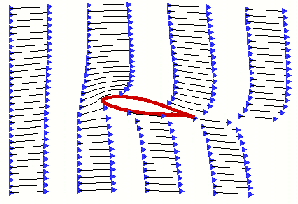Fig 2 Simulation of the airflow over a wing in a wind tunnel, with colored "smoke" to show the acceleration and deceleration of the air.

The popular explanation also implies that inverted flight is impossible. It certainly does not address acrobatic airplanes, with symmetric wings (the top and bottom surfaces are the same shape), or how a wing adjusts for the great changes in load such as when pulling out of a dive or in a steep turn.

So, why has the popular explanation prevailed for so long? One answer is that the Bernoulli principle is easy to understand. There is nothing wrong with the Bernoulli principle, or with the statement that the air goes faster over the top of the wing. But, as the above discussion suggests, our understanding is not complete with this explanation. The problem is that we are missing a vital piece when we apply Bernoulli's principle. We can calculate the pressures around the wing if we know the speed of the air over and under the wing, but how do we determine the speed?

Another fundamental shortcoming of the popular explanation is that it ignores the work that is done. Lift requires power (which is work per time). As will be seen later, an understanding of power is key to the understanding of many of the interesting phenomena of lift.

## Newton's laws and lift

So, how does a wing generate lift? To begin to understand lift we must return to high school physics and review Newton's first and third laws. (We will introduce Newton's second law a little later.) Newton's first law states a body at rest will remain at rest, and a body in motion will continue in straight-line motion unless subjected to an external applied force. That means, if one sees a bend in the flow of air, or if air originally at rest is accelerated into motion, there is a force acting on it. Newton's third law states that for every action there is an equal and opposite reaction. As an example, an object sitting on a table exerts a force on the table (its weight) and the table puts an equal and opposite force on the object to hold it up. In order to generate lift a wing must do something to the air. What the wing does to the air is the action while lift is the reaction.

Let's compare two figures used to show streams of air (streamlines) over a wing. In figure 3 the air comes straight at the wing, bends around it, and then leaves straight behind the wing. We have all seen similar pictures, even in flight manuals. But, the air leaves the wing exactly as it appeared ahead of the wing. There is no net action on the air so there can be no lift! Figure 4 shows the streamlines, as they should be drawn. The air passes over the wing and is bent down. The bending of the air is the action. The reaction is the lift on the wing.Fig 3 Common depiction of airflow over a wing. This wing has no lift.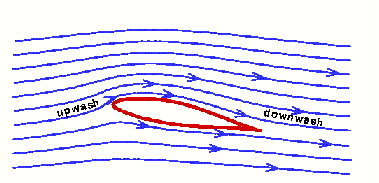Fig 4 True airflow over a wing with lift, showing upwash and downwash.

## The wing as a pump

As Newton's laws suggest, the wing must change something of the air to get lift. Changes in the air's momentum will result in forces on the wing. To generate lift a wing must divert air down, lots of air.

The lift of a wing is equal to the change in momentum of the air it diverts down. Momentum is the product of mass and velocity. The lift of a wing is proportional to the amount of air diverted down times the downward velocity of that air. Its that simple. (Here we have used an alternate form of Newton's second law that relates the acceleration of an object to its mass and to the force on it, F=ma) For more lift the wing can either divert more air (mass) or increase its downward velocity. This downward velocity behind the wing is called "downwash". Figure 5 shows how the downwash appears to the pilot (or in a wind tunnel). The figure also shows how the downwash appears to an observer on the ground watching the wing go by. To the pilot the air is coming off the wing at roughly the angle of attack. To the observer on the ground, if he or she could see the air, it would be coming off the wing almost vertically. The greater the angle of attack, the greater the vertical velocity. Likewise, for the same angle of attack, the greater the speed of the wing the greater the vertical velocity. Both the increase in the speed and the increase of the angle of attack increase the length of the vertical arrow. It is this vertical velocity that gives the wing lift.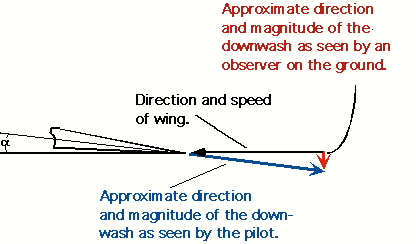Fig 5 How downwash appears to a pilot and to an observer on the ground.

As stated, an observer on the ground would see the air going almost straight down behind the plane. This can be demonstrated by observing the tight column of air behind a propeller, a household fan, or under the rotors of a helicopter, all of which are rotating wings. If the air were coming off the blades at an angle the air would produce a cone rather than a tight column. If a plane were to fly over a very large scale, the scale would register the weight of the plane.

If we estimate the average vertical component of the downwash of a Cessna 172 traveling at 110 knots to be about 9 knots, then to generate the needed 2,300 lbs of lift the wing pumps a whopping 2.5 ton/sec of air! In fact, as will be discussed later, this estimate may be as much as a factor of two too low. The amount of air pumped down for a Boeing 747 to create lift for its roughly 800,000 pounds takeoff weight is incredible indeed.

Pumping, or diverting, so much air down is a strong argument against lift being just a surface effect as implied by the popular explanation. In fact, in order to pump 2.5 ton/sec the wing of the Cessna 172 must accelerate all of the air within 9 feet above the wing. (Air weighs about 2 pounds per cubic yard at sea level.) Figure 6 illustrates the effect of the air being diverted down from a wing. A huge hole is punched through the fog by the downwash from the airplane that has just flown over it.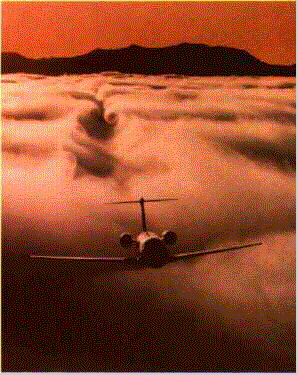Fig 6 Downwash and wing vortices in the fog. (Photographer Paul Bowen, courtesy of Cessna Aircraft, Co.)

So how does a thin wing divert so much air? When the air is bent around the top of the wing, it pulls on the air above it accelerating that air down, otherwise there would be voids in the air left above the wing. Air is pulled from above to prevent voids. This pulling causes the pressure to become lower above the wing. It is the acceleration of the air above the wing in the downward direction that gives lift. (Why the wing bends the air with enough force to generate lift will be discussed in the next section.)

As seen in figure 4, a complication in the picture of a wing is the effect of "upwash" at the leading edge of the wing. As the wing moves along, air is not only diverted down at the rear of the wing, but air is pulled up at the leading edge. This upwash actually contributes to negative lift and more air must be diverted down to compensate for it. This will be discussed later when we consider ground effect.

Normally, one looks at the air flowing over the wing in the frame of reference of the wing. In other words, to the pilot the air is moving and the wing is standing still. We have already stated that an observer on the ground would see the air coming off the wing almost vertically. But what is the air doing above and below the wing? Figure 7 shows an instantaneous snapshot of how air molecules are moving as a wing passes by. Remember in this figure the air is initially at rest and it is the wing moving. Ahead of the leading edge, air is moving up (upwash). At the trailing edge, air is diverted down (downwash). Over the top the air is accelerated towards the trailing edge. Underneath, the air is accelerated forward slightly, if at all.Fig 7 Direction of air movement around a wing as seen by an observer on the ground.

In the mathematical aerodynamics description of lift this rotation of the air around the wing gives rise to the "bound vortex" or "circulation" model. The advent of this model, and the complicated mathematical manipulations associated with it, leads to the direct understanding of forces on a wing. But, the mathematics required typically takes students in aerodynamics some time to master.

One observation that can be made from figure 7 is that the top surface of the wing does much more to move the air than the bottom. So the top is the more critical surface. Thus, airplanes can carry external stores, such as drop tanks, under the wings but not on top where they would interfere with lift. That is also why wing struts under the wing are common but struts on the top of the wing have been historically rare. A strut, or any obstruction, on the top of the wing would interfere with the lift.

## Air has viscosity

The natural question is "how does the wing divert the air down?" When a moving fluid, such as air or water, comes into contact with a curved surface it will try to follow that surface. To demonstrate this effect, hold a water glass horizontally under a faucet such that a small stream of water just touches the side of the glass. Instead of flowing straight down, the presence of the glass causes the water to wrap around the glass as is shown in figure 8. This tendency of fluids to follow a curved surface is known as the Coanda effect. From Newton's first law we know that for the fluid to bend there must be a force acting on it. From Newton's third law we know that the fluid must put an equal and opposite force on the object that caused the fluid to bend.Fig 8 Coanda effect.

Why should a fluid follow a curved surface? The answer is viscosity: the resistance to flow which also gives the air a kind of "stickiness." Viscosity in air is very small but it is enough for the air molecules to want to stick to the surface. The relative velocity between the surface and the nearest air molecules is exactly zero. (That is why one cannot hose the dust off of a car and why there is dust on the backside of the fans in a wind tunnel.) Just above the surface the fluid has some small velocity. The farther one goes from the surface the faster the fluid is moving until the external velocity is reached (note that this occurs in less than an inch). Because the fluid near the surface has a change in velocity, the fluid flow is bent towards the surface. Unless the bend is too tight, the fluid will follow the surface. This volume of air around the wing that appears to be partially stuck to the wing is called the "boundary layer".

## Lift as a function of angle of attack

There are many types of wing: conventional, symmetric, conventional in inverted flight, the early biplane wings that looked like warped boards, and even the proverbial "barn door." In all cases, the wing is forcing the air down, or more accurately pulling air down from above. What all of these wings have in common is an angle of attack with respect to the oncoming air. It is this angle of attack that is the primary parameter in determining lift. The lift of the inverted wing can be explained by its angle of attack, despite the apparent contradiction with the popular explanation involving the Bernoulli principle. A pilot adjusts the angle of attack to adjust the lift for the speed and load. The popular explanation of lift which focuses on the shape of the wing gives the pilot only the speed to adjust.

To better understand the role of the angle of attack it is useful to introduce an "effective" angle of attack, defined such that the angle of the wing to the oncoming air that gives zero lift is defined to be zero degrees. If one then changes the angle of attack both up and down one finds that the lift is proportional to the angle. Figure 9 shows the coefficient of lift (lift normalized for the size of the wing) for a typical wing as a function of the effective angle of attack. A similar lift versus angle of attack relationship is found for all wings, independent of their design. This is true for the wing of a 747 or a barn door. The role of the angle of attack is more important than the details of the airfoil's shape in understanding lift.Fig 9 Coefficient of lift versus the effective angle of attack.

Typically, the lift begins to decrease at an angle of attack of about 15 degrees. The forces necessary to bend the air to such a steep angle are greater than the viscosity of the air will support, and the air begins to separate from the wing. This separation of the airflow from the top of the wing is a stall.

## The wing as air "scoop"

We now would like to introduce a new mental image of a wing. One is used to thinking of a wing as a thin blade that slices through the air and develops lift somewhat by magic. The new image that we would like you to adopt is that of the wing as a scoop diverting a certain amount of air from the horizontal to roughly the angle of attack, as depicted in figure 10. The scoop can be pictured as an invisible structure put on the wing at the factory. The length of the scoop is equal to the length of the wing and the height is somewhat related to the chord length (distance from the leading edge of the wing to the trailing edge). The amount of air intercepted by this scoop is proportional to the speed of the plane and the density of the air, and nothing else.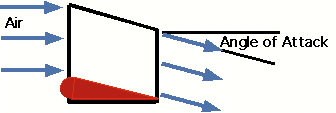Fig 10 The wing as a scoop.

As stated before, the lift of a wing is proportional to the amount of air diverted down times the vertical velocity of that air. As a plane increases speed, the scoop diverts more air. Since the load on the wing, which is the weight of the plane, does not increase the vertical speed of the diverted air must be decreased proportionately. Thus, the angle of attack is reduced to maintain a constant lift. When the plane goes higher, the air becomes less dense so the scoop diverts less air for the same speed. Thus, to compensate the angle of attack must be increased. The concepts of this section will be used to understand lift in a way not possible with the popular explanation.

## Lift requires power

When a plane passes overhead the formerly still air ends up with a downward velocity. Thus, the air is left in motion after the plane leaves. The air has been given energy. Power is energy, or work, per time. So, lift must require power. This power is supplied by the airplane's engine (or by gravity and thermals for a sailplane).

How much power will we need to fly? The power needed for lift is the work (energy) per unit time and so is proportional to the amount of air diverted down times the velocity squared of that diverted air. We have already stated that the lift of a wing is proportional to the amount of air diverted down times the downward velocity of that air. Thus, the power needed to lift the airplane is proportional to the load (or weight) times the vertical velocity of the air. If the speed of the plane is doubled the amount of air diverted down doubles. Thus the angle of attack must be reduced to give a vertical velocity that is half the original to give the same lift. The power required for lift has been cut in half. This shows that the power required for lift becomes less as the airplane's speed increases. In fact, we have shown that this power to create lift is proportional to one over the speed of the plane.

But, we all know that to go faster (in cruise) we must apply more power. So there must be more to power than the power required for lift. The power associated with lift, described above, is often called the "induced" power. Power is also needed to overcome what is called "parasitic" drag, which is the drag associated with moving the wheels, struts, antenna, etc. through the air. The energy the airplane imparts to an air molecule on impact is proportional to the speed squared. The number of molecules struck per time is proportional to the speed. Thus the parasitic power required to overcome parasitic drag increases as the speed cubed.

Figure 11 shows the power curves for induced power, parasitic power, and total power which is the sum of induced power and parasitic power. Again, the induced power goes as one over the speed and the parasitic power goes as the speed cubed. At low speed the power requirements of flight are dominated by the induced power. The slower one flies the less air is diverted and thus the angle of attack must be increased to maintain lift. Pilots practice flying on the "backside of the power curve" so that they recognize that the angle of attack and the power required to stay in the air at very low speeds are considerable.Fig 11 Power requirements versus speed.

At cruise, the power requirement is dominated by parasitic power. Since this goes as the speed cubed an increase in engine size gives one a faster rate of climb but does little to improve the cruise speed of the plane.

Since we now know how the power requirements vary with speed, we can understand drag, which is a force. Drag is simply power divided by speed. Figure 12 shows the induced, parasitic, and total drag as a function of speed. Here the induced drag varies as one over speed squared and parasitic drag varies as the speed squared. Taking a look at these curves one can deduce a few things about how airplanes are designed. Slower airplanes, such as gliders, are designed to minimize induced drag (or induced power), which dominates at lower speeds. Faster airplanes are more concerned with parasitic drag (or parasitic power).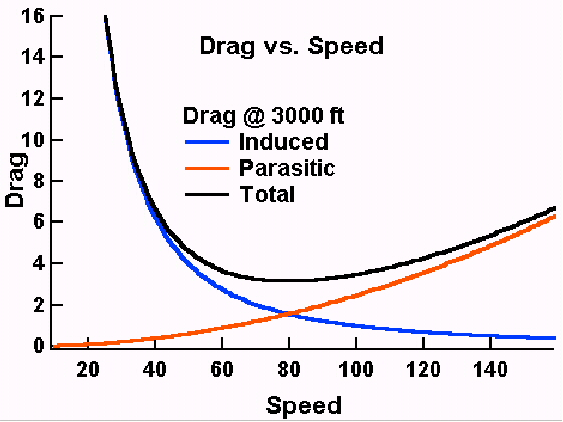Fig 12 Drag versus speed.

## Wing efficiency

At cruise, a non-negligible amount of the drag of a modern wing is induced drag. Parasitic drag, which dominates at cruise, of a Boeing 747 wing is only equivalent to that of a 1/2-inch cable of the same length. One might ask what affects the efficiency of a wing. We saw that the induced power of a wing is proportional to the vertical velocity of the air. If the length of a wing were to be doubled, the size of our scoop would also double, diverting twice as much air. So, for the same lift the vertical velocity (and thus the angle of attack) would have to be halved. Since the induced power is proportional to the vertical velocity of the air, it too is reduced by half. Thus, the lifting efficiency of a wing is proportional to one over the length of the wing. The longer the wing the less induced power required to produce the same lift, though this is achieved with an increase in parasitic drag. Low speed airplanes are affected more by induced drag than fast airplanes and so have longer wings. That is why sailplanes, which fly at low speeds, have such long wings. High-speed fighters, on the other hand, feel the effects of parasitic drag more than our low speed trainers. Therefore, fast airplanes have shorter wings to lower parasite drag.

There is a misconception held by some that lift does not require power. This comes from aeronautics in the study of the idealized theory of wing sections (airfoils). When dealing with an airfoil, the picture is actually that of a wing with infinite span. Since we have seen that the power necessary for lift is proportional to one over the length of the wing, a wing of infinite span does not require power for lift. If lift did not require power airplanes would have the same range full as they do empty, and helicopters could hover at any altitude and load. Best of all, propellers (which are rotating wings) would not require power to produce thrust. Unfortunately, we live in the real world where both lift and propulsion require power.

Let us now consider the relationship between wing loading and power. Does it take more power to fly more passengers and cargo? And, does loading affect stall speed? At a constant speed, if the wing loading is increased the vertical velocity must be increased to compensate. This is done by increasing the angle of attack. If the total weight of the airplane were doubled (say, in a 2-g turn) the vertical velocity of the air is doubled to compensate for the increased wing loading. The induced power is proportional to the load times the vertical velocity of the diverted air, which have both doubled. Thus the induced power requirement has increased by a factor of four! The same thing would be true if the airplane's weight were doubled by adding more fuel, etc.

One way to measure the total power is to look at the rate of fuel consumption. Figure 13 shows the fuel consumption versus gross weight for a large transport airplane traveling at a constant speed (obtained from actual data). Since the speed is constant the change in fuel consumption is due to the change in induced power. The data are fitted by a constant (parasitic power) and a term that goes as the load squared. This second term is just what was predicted in our Newtonian discussion of the effect of load on induced power.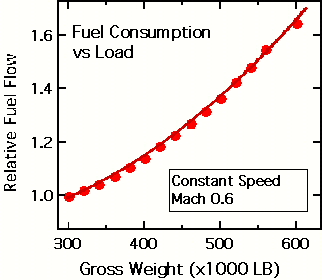Fig 13 Fuel consumption versus load for a large transport airplane traveling at a constant speed.

The increase in the angle of attack with increased load has a downside other than just the need for more power. As shown in figure 9 a wing will eventually stall when the air can no longer follow the upper surface, that is, when the critical angle is reached. Figure 14 shows the angle of attack as a function of airspeed for a fixed load and for a 2-g turn. The angle of attack at which the plane stalls is constant and is not a function of wing loading. The stall speed increases as the square root of the load. Thus, increasing the load in a 2-g turn increases the speed at which the wing will stall by 40%. An increase in altitude will further increase the angle of attack in a 2-g turn. This is why pilots practice "accelerated stalls" which illustrate that an airplane can stall at any speed. For any speed there is a load that will induce a stall.Fig 14 Angle of attack versus speed for straight and level flight and for a 2-g turn.

## Wing vortices

One might ask what the downwash from a wing looks like. The downwash comes off the wing as a sheet and is related to the details of the load distribution on the wing. Figure 15 shows, through condensation, the distribution of lift on an airplane during a high-g maneuver. From the figure one can see that the distribution of load changes from the root of the wing to the tip. Thus, the amount of air in the downwash must also change along the wing. The wing near the root is "scooping" up much more air than the tip. Since the root is diverting so much air the net effect is that the downwash sheet will begin to curl outward around itself, just as the air bends around the top of the wing because of the change in the velocity of the air. This is the wing vortex. The tightness of the curling of the wing vortex is proportional to the rate of change in lift along the wing. At the wing tip the lift must rapidly become zero causing the tightest curl. This is the wing tip vortex and is just a small (though often most visible) part of the wing vortex. Returning to figure 6 one can clearly see the development of the wing vortices in the downwash as well as the wing tip vortices.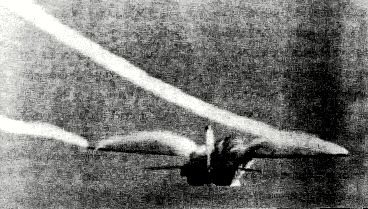Fig 15 Condensation showing the distribution of lift along a wing. The wingtip vortices are also seen. (from Patterns in the Sky, J.F. Campbell and J.R. Chambers, NASA SP-514.)

Winglets (those small vertical extensions on the tips of some wings) are used to improve the efficiency of the wing by increasing the effective length of the wing. The lift of a normal wing must go to zero at the tip because the bottom and the top communicate around the end. The winglets blocks this communication so the lift can extend farther out on the wing. Since the efficiency of a wing increases with length, this gives increased efficiency. One caveat is that winglet design is tricky and winglets can actually be detrimental if not properly designed.

## Ground effect

Another common phenomenon that is misunderstood is that of ground effect. That is the increased efficiency of a wing when flying within a wing length of the ground. A low-wing airplane will experience a reduction in drag by 50% just before it touches down. There is a great deal of confusion about ground effect. Many pilots (and the FAA VFR Exam-O-Gram No. 47) mistakenly believe that ground effect is the result of air being compressed between the wing and the ground.

To understand ground effect it is necessary to have an understanding of upwash. For the pressures involved in low speed flight, air is considered to be non-compressible. When the air is accelerated over the top of the wing and down, it must be replaced. So some air must shift around the wing (below and forward, and then up) to compensate, similar to the flow of water around a canoe paddle when rowing. This is the cause of upwash.

As stated earlier, upwash is accelerating air in the wrong direction for lift. Thus a greater amount of downwash is necessary to compensate for the upwash as well as to provide the necessary lift. Thus more work is done and more power required. Near the ground the upwash is reduced because the ground inhibits the circulation of the air under the wing. So less downwash is necessary to provide the lift. The angle of attack is reduced and so is the induced power, making the wing more efficient.

Earlier, we estimated that a Cessna 172 flying at 110 knots must divert about 2.5 ton/sec to provide lift. In our calculations we neglected the upwash. From the magnitude of ground effect, it is clear that the amount of air diverted is probably more like 5 ton/sec.

## Conclusions

Let us review what we have learned and get some idea of how the physical description has given us a greater ability to understand flight. First what have we learned:

• The amount of air diverted by the wing is proportional to the speed of the wing and the air density.
• The vertical velocity of the diverted air is proportional to the speed of the wing and the angle of attack.
• The lift is proportional to the amount of air diverted times the vertical velocity of the air.
• The power needed for lift is proportional to the lift times the vertical velocity of the air.

Now let us look at some situations from the physical point of view and from the perspective of the popular explanation.

• The plane's speed is reduced. The physical view says that the amount of air diverted is reduced so the angle of attack is increased to compensate. The power needed for lift is also increased. The popular explanation cannot address this.
• The load of the plane is increased. The physical view says that the amount of air diverted is the same but the angle of attack must be increased to give additional lift. The power needed for lift has also increased. Again, the popular explanation cannot address this.
• A plane flies upside down. The physical view has no problem with this. The plane adjusts the angle of attack of the inverted wing to give the desired lift. The popular explanation implies that inverted flight is impossible.

As one can see, the popular explanation, which fixates on the shape of the wing, may satisfy many but it does not give one the tools to really understand flight. The physical description of lift is easy to understand and much more powerful.